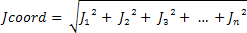# Accel. Jerk

LabVIEW 2018 SoftMotion Module

Edition Date: March 2018

Part Number: 371093W-01

»View Product InfoDownload Help (Windows Only)

Specifies the maximum acceleration jerk of the move in Units/s3. Jerk is the derivative of acceleration (change of acceleration per unit time). Setting acceleration jerk to 0 (default) allows SoftMotion to automatically calculate the jerk using the specified Smoothing. If you specify a value for acceleration jerk but not deceleration jerk SoftMotion assumes equal values for acceleration and deceleration when calculating the move profile. If you specify jerk values but not acceleration and deceleration values, SoftMotion automatically calculates all values and uses the provided jerk values as maximum limits.

Decreasing the acceleration jerk smooths the acceleration portion of the motion profile, resulting in less abrupt transitions from start motion to acceleration and acceleration to constant velocity. Coordinate acceleration jerk is calculated using the root sum of squares as follows:The following figure shows the result of changing the acceleration jerk on the motion profile:A sufficiently low jerk will smooth the profile so much that the maximum acceleration value is never reached.

 Generating Profiles Performing a Move When Calculated When Solve Profile is called. During move execution. Other Influencing Factors length of moveother move constraint values (velocity, acceleration, deceleration, and jerk) length of moveother move constraint values (velocity, acceleration, deceleration, and jerk)PID tuning parametersphysical constraints of the drive/motor/mechanical system